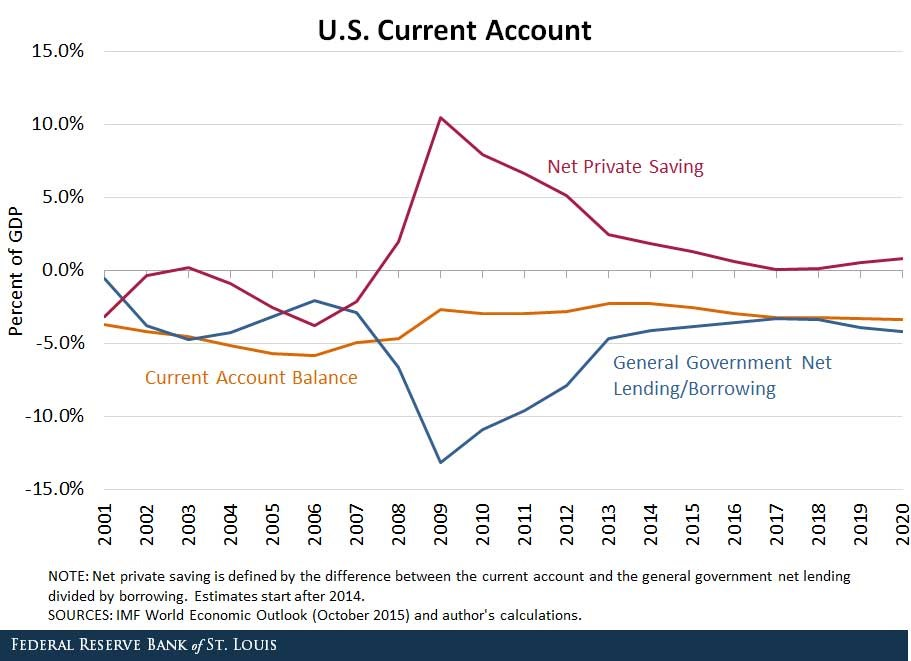# Fight Finance

#### CoursesTagsRandomAllRecentScores

For a price of $1040, Camille will sell you a share which just paid a dividend of$100, and is expected to pay dividends every year forever, growing at a rate of 5% pa.

So the next dividend will be $100(1+0.05)^1=105.00$, and the year after it will be $100(1+0.05)^2=110.25$ and so on.

The required return of the stock is 15% pa.

Would you like to the share or politely ?

A three year bond has a fixed coupon rate of 12% pa, paid semi-annually. The bond's yield is currently 6% pa. The face value is $100. What is its price? Your friend just bought a house for$1,000,000. He financed it using a $900,000 mortgage loan and a deposit of$100,000.

In the context of residential housing and mortgages, the 'equity' or 'net wealth' tied up in a house is the value of the house less the value of the mortgage loan. Assuming that your friend's only asset is his house, his net wealth is $100,000. If house prices suddenly fall by 15%, what would be your friend's percentage change in net wealth? Assume that: • No income (rent) was received from the house during the short time over which house prices fell. • Your friend will not declare bankruptcy, he will always pay off his debts. If the current AUD exchange rate is USD 0.9686 = AUD 1, what is the American terms quote of the AUD against the USD? In the Merton model of corporate debt, buying a levered company's shares is equivalent to: A trader sells one crude oil European style call option contract on the CME expiring in one year with an exercise price of$44 per barrel for a price of $6.64. The crude oil spot price is$40.33. If the trader doesn’t close out her contract before maturity, then at maturity she will have the:

A one year European-style call option has a strike price of $4. The option's underlying stock pays no dividends and currently trades at$5. The risk-free interest rate is 10% pa continuously compounded. Use a single step binomial tree to calculate the option price, assuming that the price could rise to $8 $(u = 1.6)$ or fall to$3.125 $(d = 1/1.6)$ in one year. The call option price now is:

The market expects the Reserve Bank of Australia (RBA) to increase the policy rate by 25 basis points at their next meeting. The current exchange rate is 0.8 USD per AUD.

Then unexpectedly, the RBA announce that they will increase the policy rate by 50 basis points due to increased fears of inflation.

What do you expect to happen to Australia's exchange rate on the day when the surprise announcement is made? The Australian dollar is likely to suddenly:

Observe the below graph of the US current account surplus as a proportion of GDP.Define lending as buying (or saving or investing in) debt and equity assets.

The sum of US ‘net private saving’ plus ‘net general government lending’ equals the US:

A hedge fund issued zero coupon bonds with a combined $1 billion face value due to be paid in 3 years. The promised yield to maturity is currently 6% pa given as a continuously compounded return (or log gross discrete return, $LGDR=\ln[P_T/P_0] \div T$). The hedge fund owns stock assets worth$1.1 billion now which are expected to have a 10% pa arithmetic average log gross discrete return $(\text{AALGDR} = \sum\limits_{t=1}^T{\left( \ln[P_t/P_{t-1}] \right)} \div T)$ and 30pp pa standard deviation (SDLGDR) in the future.

Analyse the hedge fund using the Merton model of corporate equity as an option on the firm's assets.

The risk free government bond yield to maturity is currently 5% pa given as a continuously compounded return or LGDR.

Which of the below statements is NOT correct? All figures are rounded to the sixth decimal place.